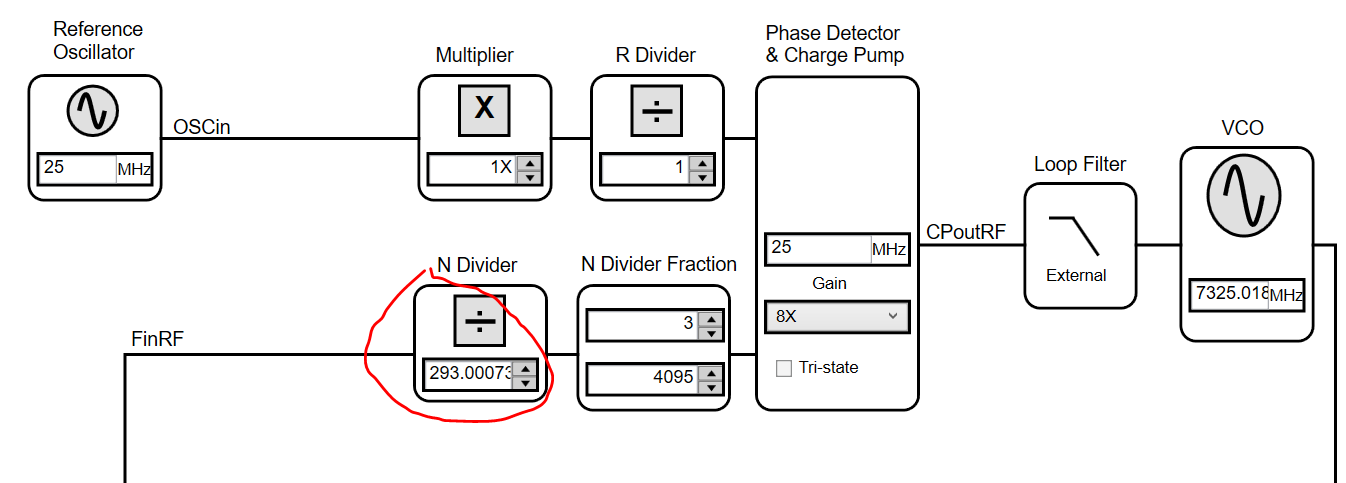If you have a related question, please click the "Ask a related question" button in the top right corner. The newly created question will be automatically linked to this question.

# LMX2487E: Need a formula sheet or App Note to show how to set registers

Part Number: LMX2487E
Other Parts Discussed in Thread: LMX2485, LMX2487

First of all, the TICS Pro GUI is fantastic and a primary reason why we always use TI PLLs.  Thanks for making that so useful.

Now I'm trying to write my own driver for embedded control.  I've asked Dean before for source behind TICS, and have found that it is written in IronPython.  Unfortunately some of the boards are set up by configuration files, like the LMX2487E, and I don't see where the underlying register-calculating python script is.

Is there some example guide or formula sheet for programming the LMX2487E PLL?  In some datasheets there are worked examples but not for the LMX2487E.  I would like to let the user provide a target LO and have the driver functions set up the registers to enable/disable IF vs. RF based on the frequency region.  Then, like TICS, it should adjust the N, FN, FD, ... values to achieve the desired frequency.  Is there an App Note showing how to determine the algorithm to set these values?

Thanks,
Darren

• Hi Darren,

Please find the python script and config files for LMX2487E here: LMX2487E.zip

You can refer to the script on how to set N divider, PFD and so on when frequency changes.

Regards,
Hao

• Ok, this is a nice start.  I went and looked at the LMX2485_PLL.py.  It is a helpful place to build from.  Now I have some follow ups.

1) First, in the 2487E datasheet is there a typo in Table 19?  Shouldn't the max frequency for this part go up to 7500M?  I want to use the 7000 - 7500 range, so I'm happy fixing RF_P = 1, but of course only if the max frequency goes to 7500M.

2) Second question.  Can you explain a bit more how the N Divider is set.  I don't understand where the RF_A, RF_B counters come into play.  Further, how can the N Divider have a decimal value if it is represented by 10 bits?Per discussions in 8.3.8, I am happy to keep the RF_FD value at 4095, to use as large a denominator as possible but not have to use the extra register bits.

So to recap, I've set the multiplier (OSC_2X=0), I think I'm happy with RF_R=1, and the RF_FD is fixed at 4095.  So what I need, in order to program a function that accepts a target LO and sets up the registers, is a calculation of RF_FN and RF_N.

73245.018 = 25 * 1 / 1  * (N + FN/4095)

3) How do I decide on these values?

Thanks,

Darren

• I am using 1.6.9.0.  That is the most up to date version on the website.  Your GUI looks quite different though.

• Darren,

1) Yes, this is a typo in the datasheet, max frequency should be 7500 MHz;

2) for the N divider value, this is a mathematically abstracted value that is the combination of RF_N, RF_FN, and RF_FD. RF_A, RF_B, and RF_C are further sub-dividers of RF_N